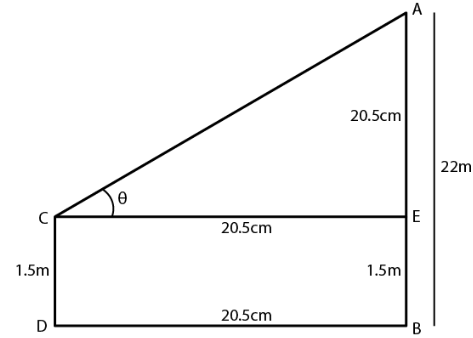Guru

# An observer 1.5 m tall is 20.5 metres away from a tower 22 metres high. Determine the angle of elevation of the top of the tower from the eye of the observer.

• 0

sir this is the question from the book -ML aggarwal( avichal publication) class 10th , chapter20 , heights and distances. IT’s very important question we have   been given that An observer 1.5 m tall is 20.5 metres away from a tower 22 metres high.
we are asked to Determine the angle of elevation of the top of the tower from the eye of the observer.

question no 13 , heights and distances , ICSE board

Share

1. In the figure,

AB is the tower and CD is an observer

θ is the angle of observationIt is given that

AB = 22m

CD = 1.5 m

Distance BD = 20.5 m

From the point C construct CE parallel tp DB

AE = 22 – 1.5 = 20.5 m

CE = DB = 20.5 m

tan θ = AE/CE

Substituting the values

tan θ = 20.5/20.5 = 1

tan 450 = 1

θ = 450

• 0